Math 333/333H

Week 2

Probability: The study of randomness and uncertainty

Relation between Probability and Statistics

Probability: Assumes properties of population are known and uses mathematics to answer questions about what we expect     to see in a sample of observations drawn from that population

Statistics: Uses sample of observations to draw conclusions about the population from which the sample was take

Experiment: An action or process that generates observations. The sample space is the set of all possible outcomes of an experiment. An event is a collection of outcomes contained in the sample space. A simple event contains a single outcome.

Use set theory to study relationships among events.

A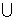B: The set containing all outcomes that are in A OR in B OR in both.

A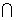B: The set containing all outcomes that are in A AND in B

A':   The set containing all outcomes that are NOT in A

S: Denotes the set containing all possible outcomes of an event.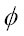: The empty set, containing no outcomes (the complement of S, S ' )

Two sets that contain no outcomes in common are mutually exclusive or disjoint

A probability is a rule assigning a numerical value to the outcome of an event.

Probability measures must obey the following rules:

1. Probability of an event, A, must be greater than or equal to zero.
2. P(S)=1
3. If we have a collection of mutually exclusive events, the probability of their union is equal to the sum of their individual probabilities.

The probability of an event, A, can be interpreted as the proportion of times that event A would occur if the same experiment was carried out over and over again. (limiting relative frequency).

1. P(A') = 1- P(A)

 2.  If A and B are mutually exclusive,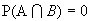.
 3. Additive law of probability: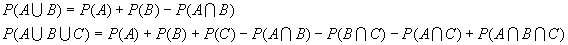If any simple event in an experiment is equally likely, then a reasonable assignment of probabilities is to let the probability of a simple event be 1/N, where N is the total number of simple events.